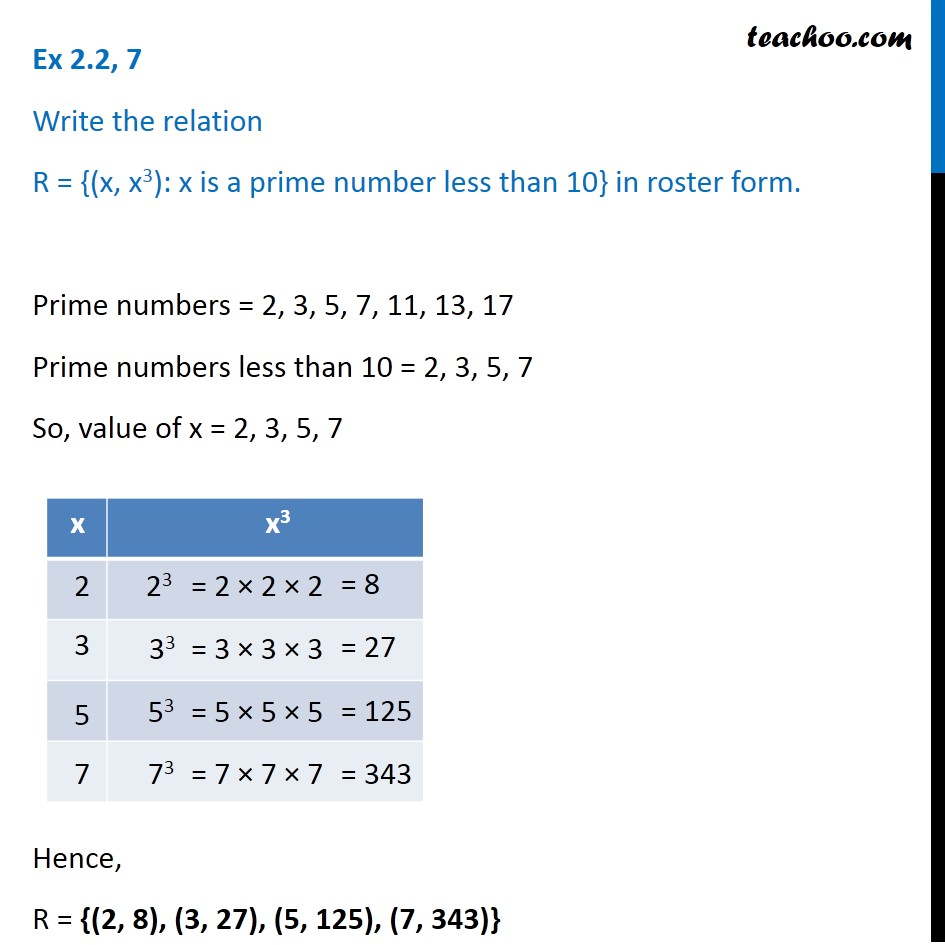Finding Relation - Set-builder form given

Chapter 2 Class 11 Relations and Functions
Concept wiseLearn in your speed, with individual attention - Teachoo Maths 1-on-1 Class

### Transcript

Ex 2.2, 7 Write the relation R = {(x, x3): x is a prime number less than 10} in roster form. Prime numbers = 2, 3, 5, 7, 11, 13, 17 Prime numbers less than 10 = 2, 3, 5, 7 So, value of x = 2, 3, 5, 7 Hence, R = {(2, 8), (3, 27), (5, 125), (7, 343)}